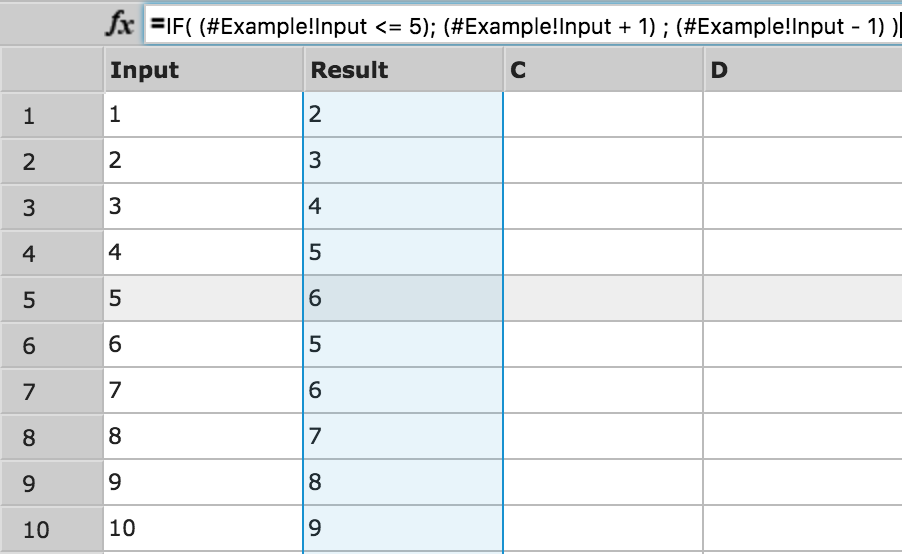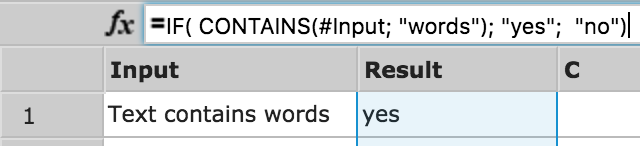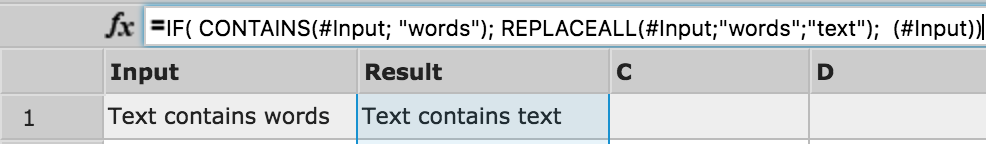How to Use the IF function for an IF THEN ELSE Statement

Goal

Learn how to use the IF function to create an IF THEN ELSE statement.

Learn

The IF function has the following syntax:

`IF( <this is #true>; <then #do()>; <else #do()>) `

Example 1

`IF( (#Example!Input <= 5); (#Example!Input + 1) ; (#Example!Input - 1) )`Example 2

`IF(CONTAINS(#Input; "words"); "yes";  "no")`Example 3

`IF( CONTAINS(#Input; "words"); REPLACEALL(#Input; "words"; "text");  (#Input))`Further Information

Please have also a look into our Community thread How can I 'pipe' or 'join' multiple formulas so I can do several IF statements?.